# Building a Simple Neural Network in R Programming

• Last Updated : 20 Aug, 2020

The term Neural Networks refers to the system of neurons either organic or artificial in nature. In artificial intelligence reference, neural networks are a set of algorithms that are designed to recognize a pattern like a human brain. They interpret sensory data through a kind of machine perception, labeling, or clustering raw input. The recognition is numerical, which is stored in vectors, into which all real-world data, be it images, sound, text, or time series, must be translated. A neural network can be pictured as a system that consists of a number of highly interconnected nodes, called ‘neurons’, which are organized in layers that process information using dynamic state responses to external inputs. Before understanding the working and architecture of neural networks, let us try to understand what artificial neurons actually are.

#### Artificial Neurons

Perceptron: Perceptrons are a type of artificial neurons developed in the 1950s and 1960s by the scientist Frank Rosenbalt, inspired by earlier work by Warren McCulloch and Walter Pitts. So, how do perceptron works? A perceptron takes several binary outputs x1, x2, …., and produces a single binary output.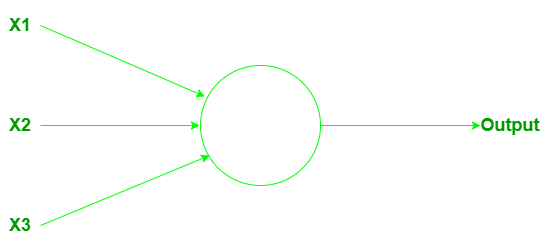It could have more or fewer inputs. To calculate/compute the output weights play an important role. Weights w1, w2, …., are real numbers expressing the importance of the respective inputs to the outputs. The neuron’s output(o or 1) totally depends upon a threshold value and is computed according to the function:Here t0 is the threshold value. It is a real number which is a parameter of the neuron. That’s the basic mathematical model. The perceptron is that it’s a device that makes decisions by weighing up the evidence. By varying the weights and the threshold, we can get different models of decision-making.

Sigmoid Neurons: Sigmoid neurons are very much closer to perceptrons, but modified so that small changes in their weights and bias cause only a small change in their output. It will allow a network of sigmoid neurons to learn more efficiently. Just like a perceptron, the sigmoid neuron has inputs, x1, x2, …. But instead of being just 0 or 1, these inputs can also be any value between 0 and 1. So, for instance, 0.567… is a valid input for a sigmoid neuron. A sigmoid neuron also has weights for each input, w1, w2, …, and an overall bias, b. But the output is not 0 or 1. Instead, it’s σ(w.x + b), where σ is called the sigmoid function:The output of a sigmoid neuron with inputs x1, x2, …, weights w1, w2, …, and bias b is:#### The Architecture of Neural Networks

A neural network consists of three layers:

1. Input Layer: Layers that take inputs based on existing data.
2. Hidden Layer: Layers that use backpropagation to optimise the weights of the input variables in order to improve the predictive power of the model.
3. Output Layer: Output of predictions based on the data from the input and hidden layers.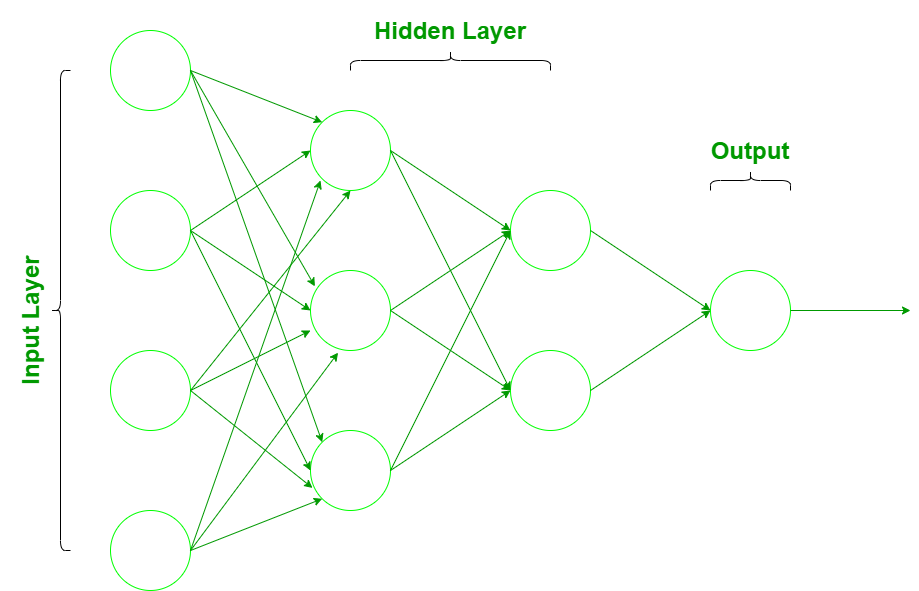The input data is introduced to the neural network through the input layer that has one neuron for each component present in the input data and is communicated to hidden layers(one or more) present in the network. It is called ‘hidden’ only because they do not constitute the input or output layer. In the hidden layers, all the processing actually happens through a system of connections characterized by weights and biases(as discussed earlier). Once the input is received, the neuron calculates a weighted sum adding also the bias and according to the result and an activation function (the most common one is sigmoid), it decides whether it should be ‘fired’ or ‘activated’. Then, the neuron transmits the information downstream to other connected neurons in a process called ‘forward pass’. At the end of this process, the last hidden layer is linked to the output layer which has one neuron for each possible desired output.

#### Implementing Neural Network in R Programming

It is very much easier to implement a neural network by using the R language because of its excellent libraries inside it. Before implementing a neural network in R let’s understand the structure of the data first.

Understanding the structure of the data

Here let’s use the binary datasets. The objective is to predict whether a candidate will get admitted to a university with variables such as gre, gpa, and rank. The R script is provided side by side and is commented for better understanding of the user. The data is in .csv format. We will get the working directory with getwd() function and place out datasets binary.csv inside it to proceed further. Please download the csv file here.

 # preparing the datasetgetwd()data <- read.csv("binary.csv" )str(data)

Output:

'data.frame':   400 obs. of  4 variables:
$admit: int 0 1 1 1 0 1 1 0 1 0 ...$ gre  : int  380 660 800 640 520 760 560 400 540 700 ...
$gpa : num 3.61 3.67 4 3.19 2.93 3 2.98 3.08 3.39 3.92 ...$ rank : int  3 3 1 4 4 2 1 2 3 2 ...


Looking at the structure of the datasets we can observe that it has 4 variables, where admit tells whether a candidate will get admitted or not admitted (1 if admitted and 0 if not admitted) gre, gpa and rank give the candidates gre score, his/her gpa in the previous college and previous college rank respectively.   We use admit as the dependent variable and gre, gpa, and rank as the independent variables. Now understand the whole process in a stepwise manner

Step 1: Scaling of the data

To set up a neural network to a dataset it is very important that we ensure a proper scaling of data. The scaling of data is essential because otherwise, a variable may have a large impact on the prediction variable only because of its scale. Using unscaled data may lead to meaningless results. The common techniques to scale data are min-max normalization, Z-score normalization, median and MAD, and tan-h estimators. The min-max normalization transforms the data into a common range, thus removing the scaling effect from all the variables. Here we are using min-max normalization for scaling data.

 # Draw a histogram for gre datahist(data$gre) Output: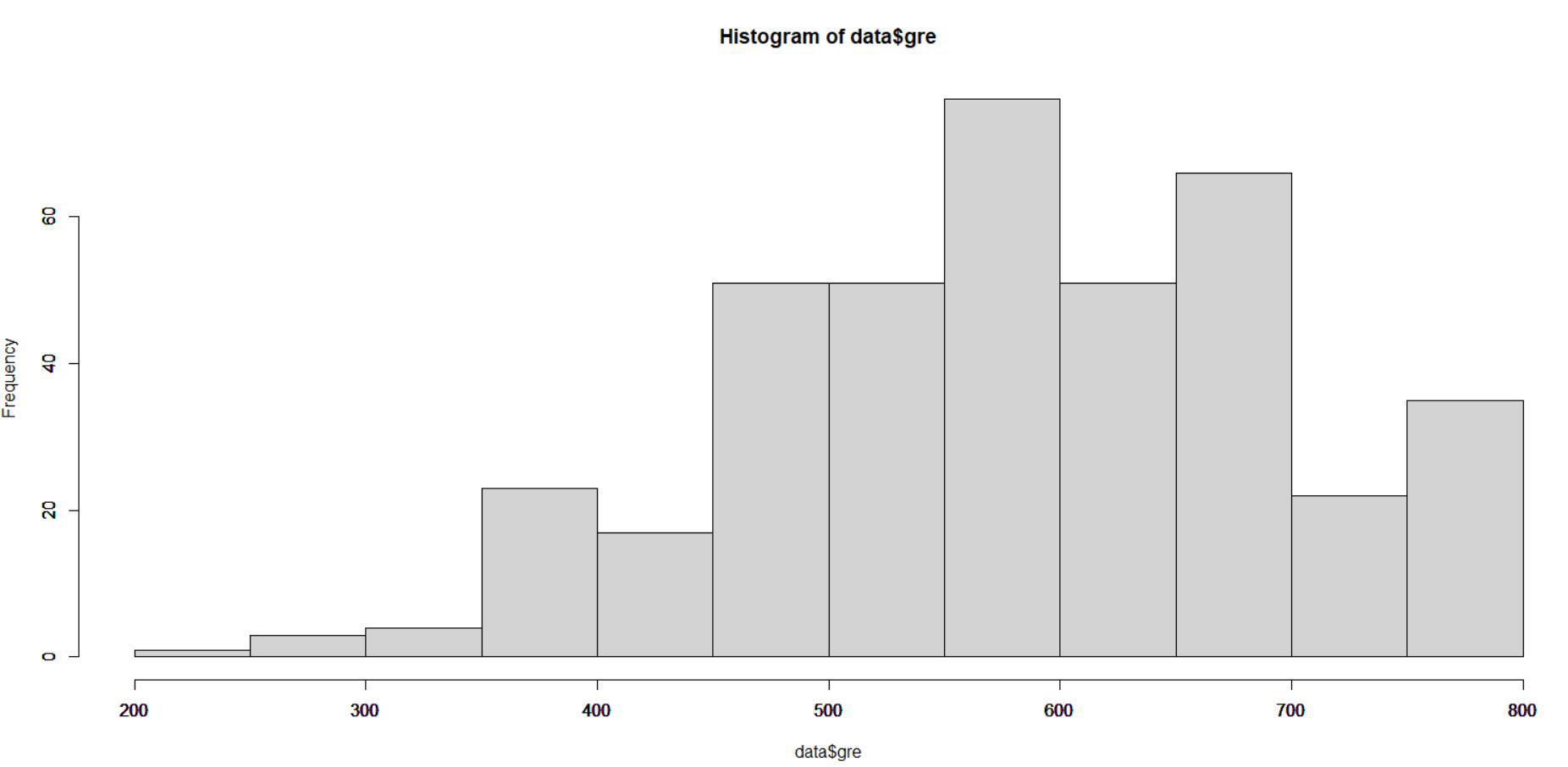From the above histogram of gre we can see that the gre varies from 200 to 800. We invoke the following function to normalize our data: normalize <- function(x) { return ((x - min(x)) / (max(x) - min(x))) }   # Min-Max Normalizationdata$gre <- (data$gre - min(data$gre)) / (max(data$gre) - min(data$gre))hist(data$gre) Output: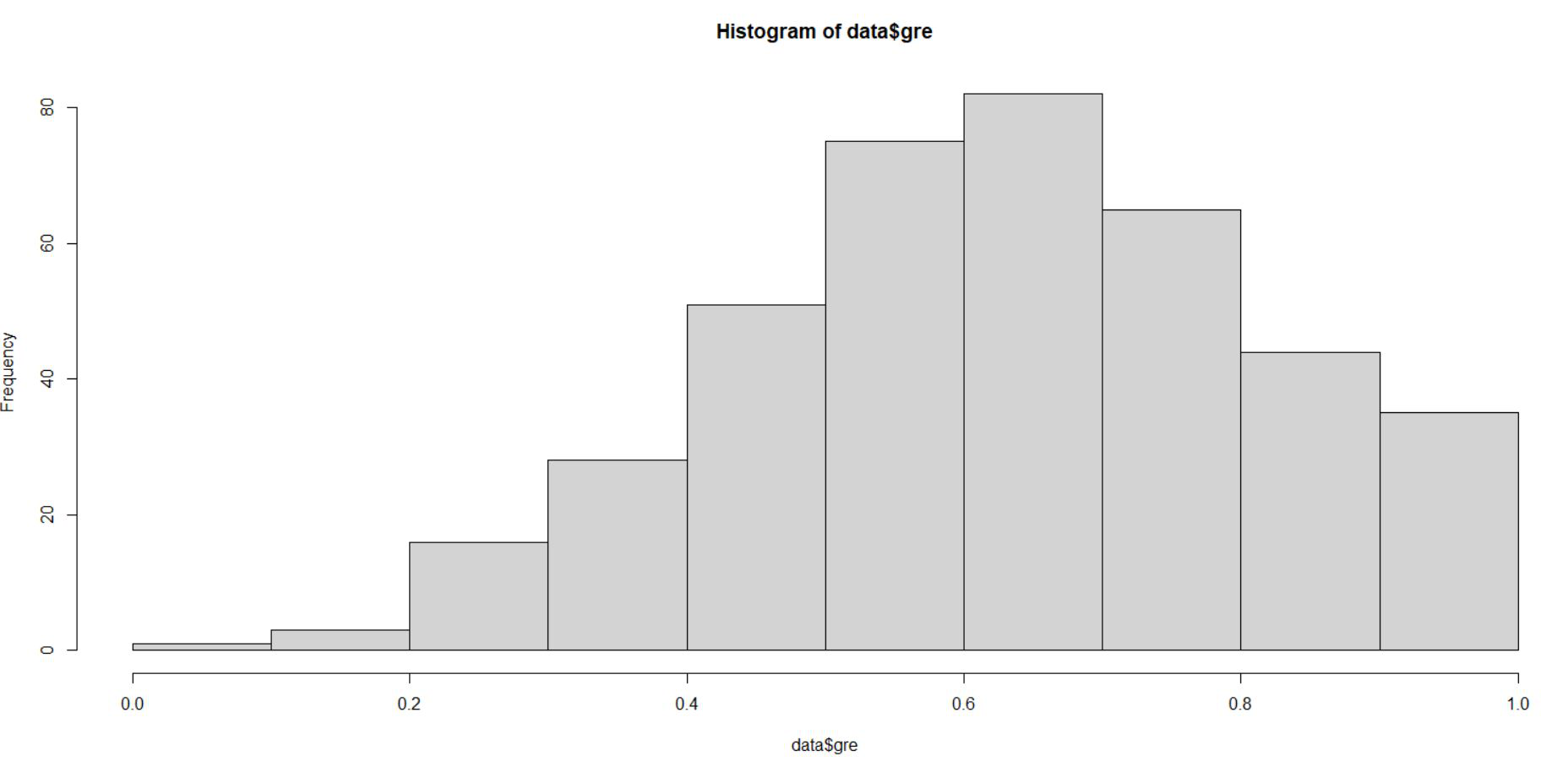From the above representation we can see that gre data is scaled in the range of 0 to 1. Similar we do for gpa and rank.  # Min-Max Normalizationdata$gpa <- (data$gpa - min(data$gpa)) / (max(data$gpa) - min(data$gpa))hist(data$gpa)data$rank <- (data$rank - min(data$rank)) / (max(data$rank) - min(data$rank))hist(data$rank) Output: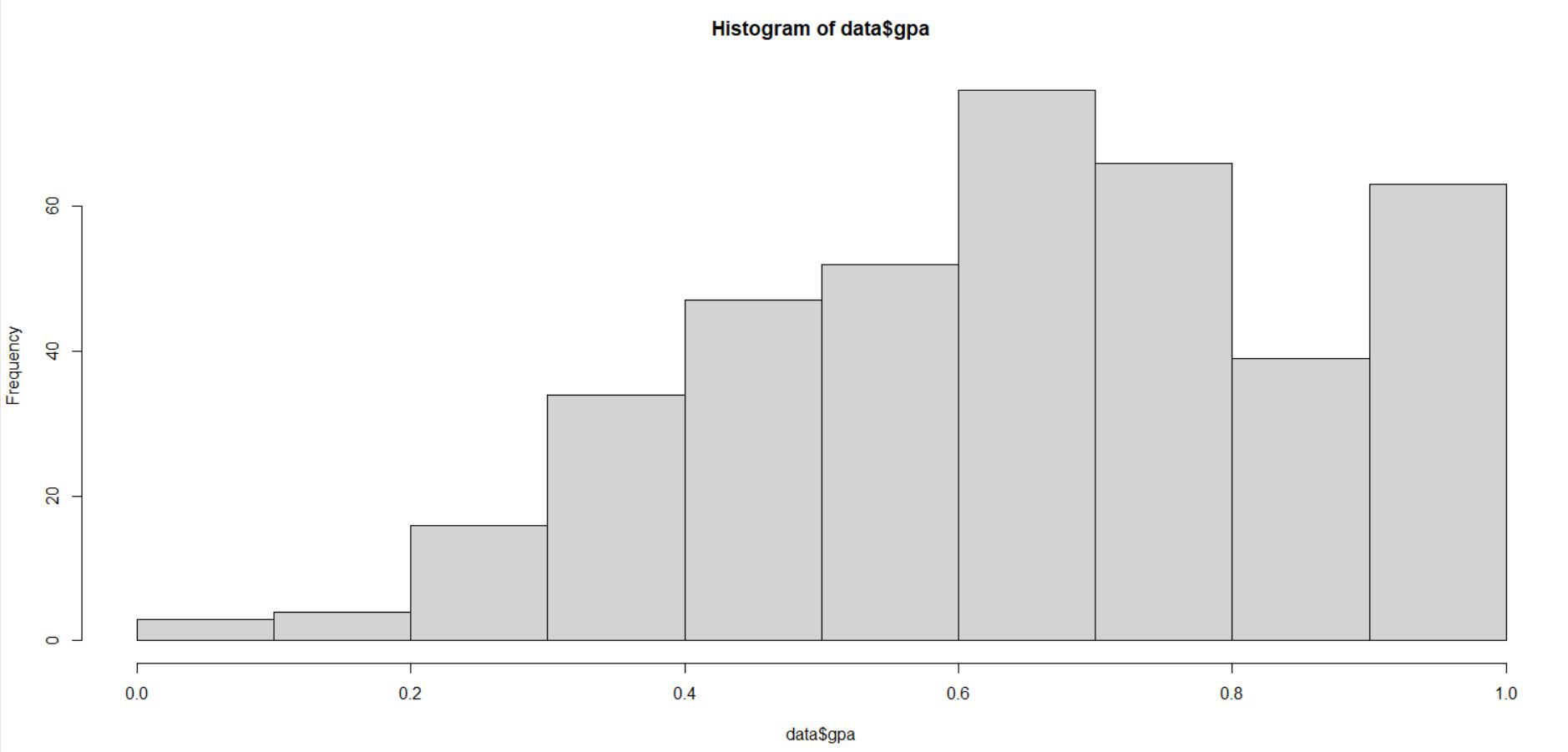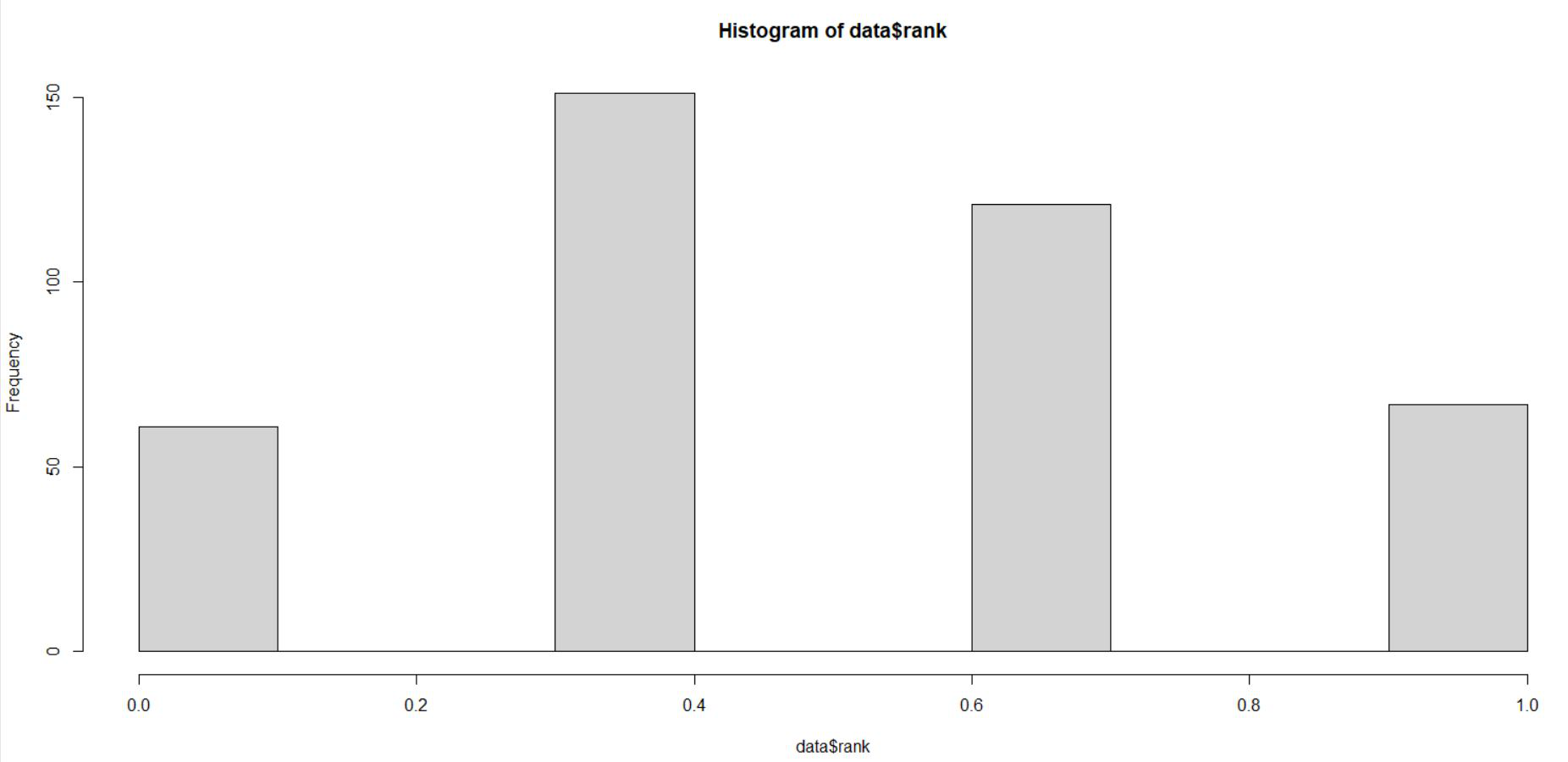It can be seen from the above two histogram representation that gpa and rank are also scaled in the range of 0 to 1. The scaled data is used to fit the neural network. Step 2: Sampling of the data Now divide the data into a training set and test set. The training set is used to find the relationship between dependent and independent variables while the test set analyses the performance of the model. We use 60% of the dataset as a training set. The assignment of the data to training and test set is done using random sampling. We perform random sampling on R using sample() function. Use set.seed()to generate same random sample every time and maintain consistency. Use the index variable while fitting neural network to create training and test data sets. The R script is as follows:  set.seed(222)inp <- sample(2, nrow(data), replace = TRUE, prob = c(0.7, 0.3))training_data <- data[inp==1, ]test_data <- data[inp==2, ] Step 3: Fitting a Neural Network Now fit a neural network on our data. We use neuralnet library for the same. neuralnet() function helps us to establish a neural network for our data. The neuralnet() function we are using here has the following syntax. Syntax: neuralnet(formula, data, hidden = 1, stepmax = 1e+05, rep = 1, lifesign = “none”, algorithm = “rprop+”, err.fct = “sse”, linear.output = TRUE) Parameters: ArgumentDescription formulaa symbolic description of the model to be fitted. dataa data frame containing the variables specified in formula. hiddena vector of integers specifying the number of hidden neurons (vertices) in each layer err.fcta differentiable function that is used for the calculation of the error. Alternatively, the strings ‘sse’ and ‘ce’ which stand for the sum of squared errors and the cross-entropy can be used. linear.outputlogical. If act.fct should not be applied to the output neurons set linear output to TRUE, otherwise to FALSE. lifesigna string specifying how much the function will print during the calculation of the neural network. ‘none’, ‘minimal’ or ‘full’. repthe number of repetitions for the neural network’s training. algorithma string containing the algorithm type to calculate the neural network. The following types are possible: ‘backprop’, ‘rprop+’, ‘rprop-‘, ‘sag’, or ‘slr’. ‘backprop’ refers to backpropagation, ‘rprop+’ and ‘rprop-‘ refer to the resilient backpropagation with and without weight backtracking, while ‘sag’ and ‘slr’ induce the usage of the modified globally convergent algorithm (grprop). stepmaxthe maximum steps for the training of the neural network. Reaching this maximum leads to a stop of the neural network’s training process.  library(neuralnet)set.seed(333)n <- neuralnet(admit~gre + gpa + rank, data = training_data, hidden = 5, err.fct = "ce", linear.output = FALSE, lifesign = 'full', rep = 2, algorithm = "rprop+", stepmax = 100000) hidden: 5 thresh: 0.01 rep: 1/2 steps: 1000 min thresh: 0.092244246452834 2000 min thresh: 0.092244246452834 3000 min thresh: 0.092244246452834 4000 min thresh: 0.092244246452834 5000 min thresh: 0.092244246452834 6000 min thresh: 0.092244246452834 7000 min thresh: 0.092244246452834 8000 min thresh: 0.0657773918077728 9000 min thresh: 0.0492128119805471 10000 min thresh: 0.0350341801886022 11000 min thresh: 0.0257113452845989 12000 min thresh: 0.0175961794629306 13000 min thresh: 0.0108791716102531 13253 error: 139.80883 time: 7.51 secs hidden: 5 thresh: 0.01 rep: 2/2 steps: 1000 min thresh: 0.147257381292693 2000 min thresh: 0.147257381292693 3000 min thresh: 0.091389043508166 4000 min thresh: 0.0648814957085886 5000 min thresh: 0.0472858320232246 6000 min thresh: 0.0359632940146351 7000 min thresh: 0.0328699898176084 8000 min thresh: 0.0305035254157369 9000 min thresh: 0.0305035254157369 10000 min thresh: 0.0241743801258625 11000 min thresh: 0.0182557959333173 12000 min thresh: 0.0136844933371039 13000 min thresh: 0.0120885410813301 14000 min thresh: 0.0109156031403791 14601 error: 147.41304 time: 8.25 secs  From the above output we conclude that both of the repetitions converge. But we will use the output-driven in the first repetition because it gives less error(139.80883) than the error(147.41304) the second repetition derives. Now, lets plot our neural network and visualize the computed neural network.  # plot our neural network plot(n, rep = 1) Output: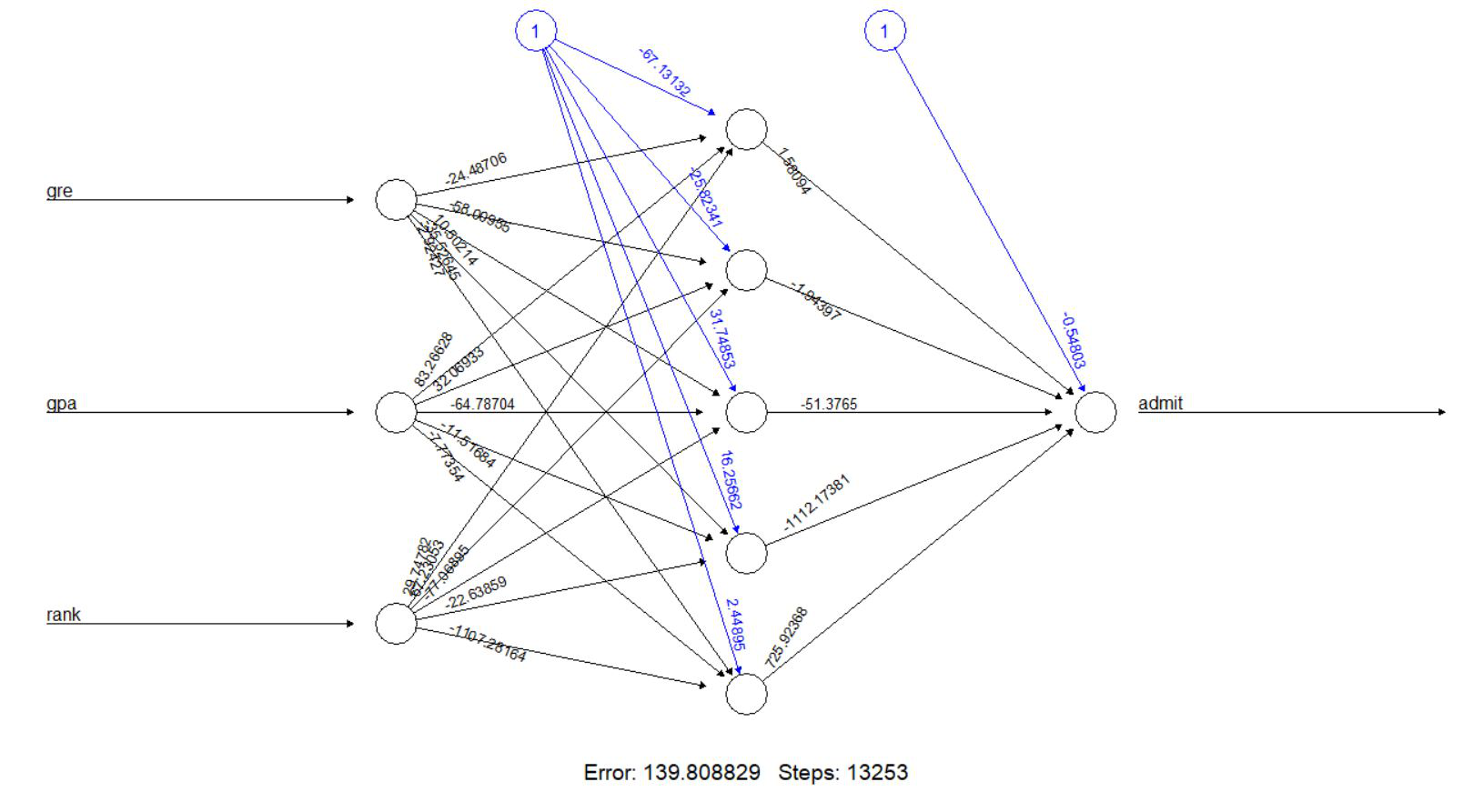The model has 5 neurons in its hidden layer. The black lines show the connections with weights. The weights are calculated using the backpropagation algorithm. The blue line is displays the bias term (constant in a regression equation). Now generate the error of the neural network model, along with the weights between the inputs, hidden layers, and outputs:  # errorn$result.matrix

Output:

                               [, 1]          [, 2]
error                  1.398088e+02  1.474130e+02
reached.threshold      9.143429e-03  9.970574e-03
steps                  1.325300e+04  1.460100e+04
Intercept.to.1layhid1 -6.713132e+01 -1.136151e+02
gre.to.1layhid1       -2.448706e+01  1.469138e+02
gpa.to.1layhid1        8.326628e+01  1.290251e+02
rank.to.1layhid1       2.974782e+01 -5.733805e+01
Intercept.to.1layhid2 -2.582341e+01  2.508958e-01
gre.to.1layhid2       -5.800955e+01  1.302115e+00
gpa.to.1layhid2        3.206933e+01 -4.856419e+00
rank.to.1layhid2       6.723053e+01  1.540390e+01
Intercept.to.1layhid3  3.174853e+01 -3.495968e+01
gre.to.1layhid3        1.050214e+01  1.325498e+02
gpa.to.1layhid3       -6.478704e+01 -4.536649e+01
rank.to.1layhid3      -7.706895e+01 -1.844943e+02
Intercept.to.1layhid4  1.625662e+01  2.188646e+01
gre.to.1layhid4       -3.552645e+01  1.956271e+01
gpa.to.1layhid4       -1.151684e+01  2.052294e+01
rank.to.1layhid4      -2.263859e+01  1.347474e+01
Intercept.to.1layhid5  2.448949e+00 -3.978068e+01
gre.to.1layhid5       -2.924269e+00 -1.569897e+02
gpa.to.1layhid5       -7.773543e+00  1.500767e+02
rank.to.1layhid5      -1.107282e+03  4.045248e+02


Step 4: Prediction

Let’s predict the rating using the neural network model. We must remember that the predicted rating will be scaled and it must be transformed in order to make a comparison with the real rating. Also compare the predicted rating with real rating.

 # Predictionoutput <- compute(n, rep = 1, training_data[, -1])head(output$net.result) Output:  [, 1] 2 0.34405929 3 0.41148373 4 0.07642387 7 0.98152454 8 0.26230256 9 0.07660906   head(training_data[1, ]) Output:  admit gre gpa rank 2 1 0.7586207 0.8103448 0.6666667  Step 5: Confusion Matrix and Misclassification error Then, we round up our results using compute() method and create a confusion matrix to compare the number of true/false positives and negatives. We will form a confusion matrix with training data  # confusion Matrix$Misclassification error -Training dataoutput <- compute(n, rep = 1, training_data[, -1])p1 <- output$net.resultpred1 <- ifelse(p1 > 0.5, 1, 0)tab1 <- table(pred1, training_data$admit)tab1

Output:

 pred1 0   1
0 177  58
1  12  34


The model generates 177 true negatives (0’s), 34 true positives (1’s), while there are 12 false negatives and 58 false positives. Now, lets calculate the misclassification error (for training data) which  {1 – classification error}

 1 - sum(diag(tab1)) / sum(tab1)

Output:

 0.2491103


The misclassification error comes out to be 24.9%. We can further increase the accuracy and efficiency of our model by increasing of decreasing nodes and bias in hidden layers .

The strength of machine learning algorithms lies in their ability to learn and improve every time in predicting an output. In the context of neural networks, it implies that the weights and biases that define the connection between neurons become more precise. This is why the weights and biases are selected such as the output from the network approximates the real value for all the training inputs. Similarly, we can make more efficient neural network models in R to predict and drive decisions.

My Personal Notes arrow_drop_up